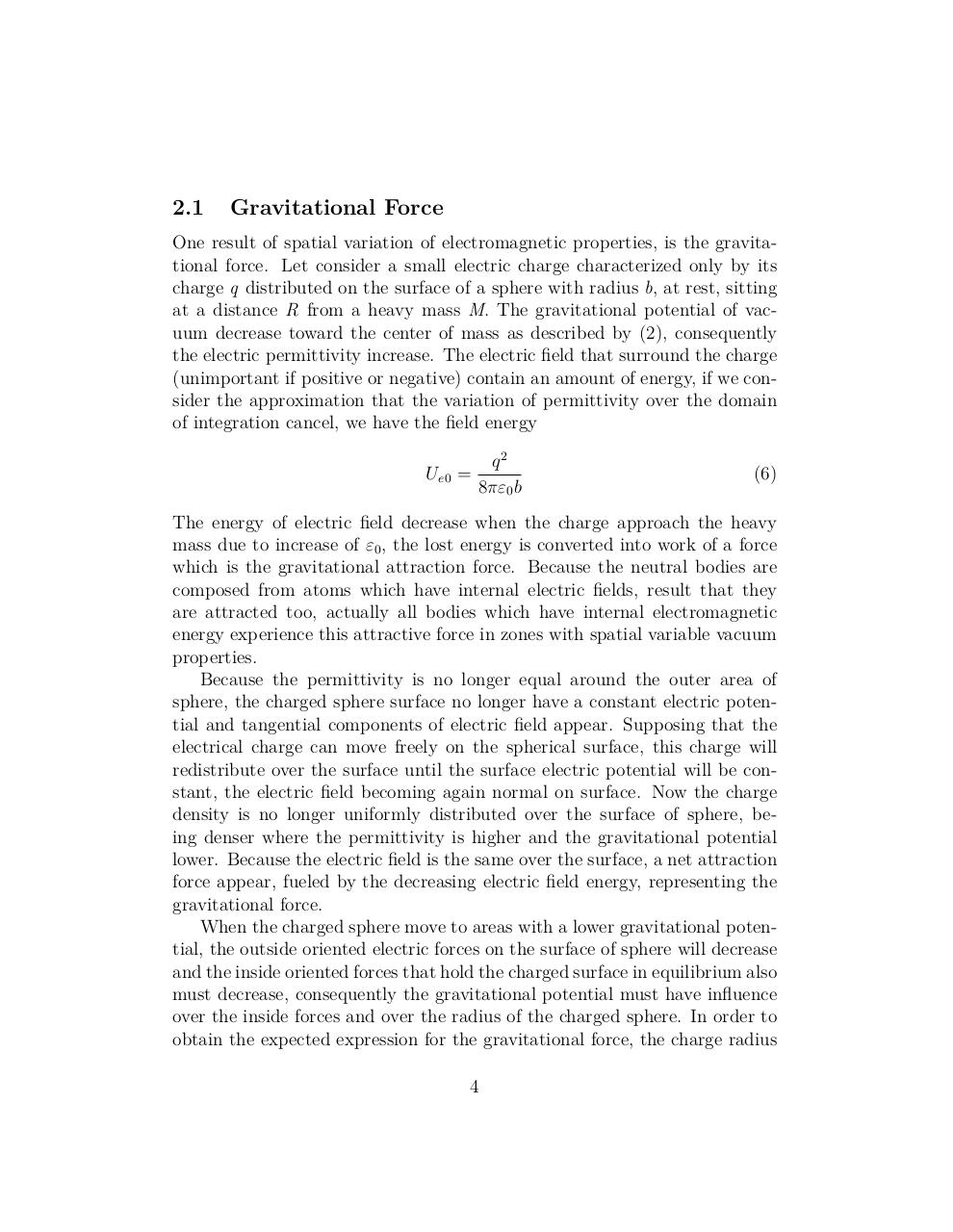# gvac art.pdfPage 1 2 3 45625

#### Text preview

2.1

Gravitational Force

One result of spatial variation of electromagnetic properties, is the gravitational force. Let consider a small electric charge characterized only by its
charge q distributed on the surface of a sphere with radius b, at rest, sitting
at a distance R from a heavy mass M. The gravitational potential of vacuum decrease toward the center of mass as described by (2), consequently
the electric permittivity increase. The electric field that surround the charge
(unimportant if positive or negative) contain an amount of energy, if we consider the approximation that the variation of permittivity over the domain
of integration cancel, we have the field energy
Ue0

q2
=
8πε0 b

(6)

The energy of electric field decrease when the charge approach the heavy
mass due to increase of ε0 , the lost energy is converted into work of a force
which is the gravitational attraction force. Because the neutral bodies are
composed from atoms which have internal electric fields, result that they
are attracted too, actually all bodies which have internal electromagnetic
energy experience this attractive force in zones with spatial variable vacuum
properties.
Because the permittivity is no longer equal around the outer area of
sphere, the charged sphere surface no longer have a constant electric potential and tangential components of electric field appear. Supposing that the
electrical charge can move freely on the spherical surface, this charge will
redistribute over the surface until the surface electric potential will be constant, the electric field becoming again normal on surface. Now the charge
density is no longer uniformly distributed over the surface of sphere, being denser where the permittivity is higher and the gravitational potential
lower. Because the electric field is the same over the surface, a net attraction
force appear, fueled by the decreasing electric field energy, representing the
gravitational force.
When the charged sphere move to areas with a lower gravitational potential, the outside oriented electric forces on the surface of sphere will decrease
and the inside oriented forces that hold the charged surface in equilibrium also
must decrease, consequently the gravitational potential must have influence
over the inside forces and over the radius of the charged sphere. In order to
obtain the expected expression for the gravitational force, the charge radius
4